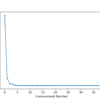# Author Archive | Stefania Cristina## The Power, Product and Quotient Rules

Optimization, as one of the core processes in many machine learning algorithms, relies on the use of derivatives in order to decide in which manner to update a model’s parameter values, to maximize or minimize an objective function.  This tutorial will continue exploring the different techniques by which we can find the derivatives of functions. […]## Derivative of the Sine and Cosine

Many machine learning algorithms involve an optimization process for different purposes. Optimization refers to the problem of minimizing or maximizing an objective function by altering the value of its inputs.  Optimization algorithms rely on the use of derivatives in order to understand how to alter (increase or decrease) the input values to the objective function, […]## What you need to know before you get started: A brief tour of Calculus Pre-Requisites

We have previously seen that calculus is one of the core mathematical concepts in machine learning that permits us to understand the internal workings of different machine learning algorithms.  Calculus, in turn, builds on several fundamental concepts that derive from algebra and geometry. The importance of having these fundamentals at hand will become even more […]## Calculus in Machine Learning: Why it Works

Calculus is one of the core mathematical concepts in machine learning that permits us to understand the internal workings of different machine learning algorithms.  One of the important applications of calculus in machine learning is the gradient descent algorithm, which, in tandem with backpropagation, allows us to train a neural network model.  In this tutorial, […]## Key Concepts in Calculus: Rate of Change

The measurement of the rate of change is an integral concept in differential calculus, which concerns the mathematics of change and infinitesimals. It allows us to find the relationship between two changing variables and how these affect one another. The measurement of the rate of change is also essential for machine learning, such as in […]## What is Calculus?

Calculus is the mathematical study of change.  The effectiveness of calculus to solve a complicated but continuous problem lies in its ability to slice the problem into infinitely simpler parts, solve them separately, and subsequently rebuild them into the original whole. This strategy can be applied to study all continuous elements that can be sliced […]## Differential Evolution from Scratch in Python

Differential evolution is a heuristic approach for the global optimisation of nonlinear and non- differentiable continuous space functions. The differential evolution algorithm belongs to a broader family of evolutionary computing algorithms. Similar to other popular direct search approaches, such as genetic algorithms and evolution strategies, the differential evolution algorithm starts with an initial population of […]